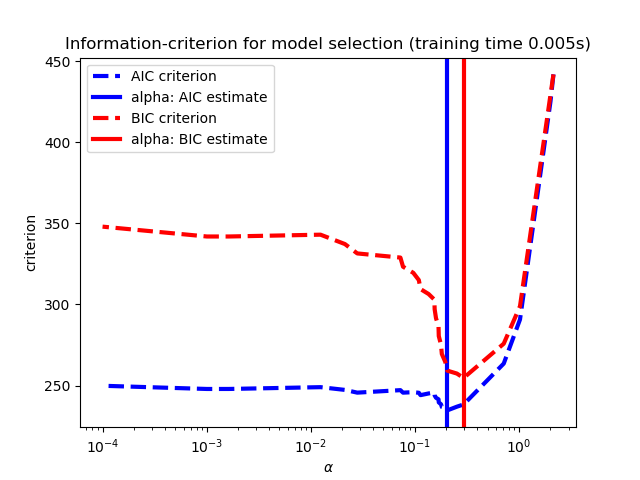# sklearn.linear_model.LassoLarsCV¶

class sklearn.linear_model.LassoLarsCV(*, fit_intercept=True, verbose=False, max_iter=500, normalize=True, precompute='auto', cv=None, max_n_alphas=1000, n_jobs=None, eps=2.220446049250313e-16, copy_X=True, positive=False)

[源码]

Lasso的优化目标是：

fit_intercept bool, default=True

verbose bool or int, default=False

max_iter int, default=500

normalize bool, default=False
fit_intercept设置为False 时，将忽略此参数。如果为True，则在回归之前通过减去均值并除以l2-范数来对回归变量X进行归一化。如果你希望标准化，请先使用 sklearn.preprocessing.StandardScaler，然后调用fit 估算器并设置normalize=False
precompute bool, ‘auto’ or array-like , default=’auto’

cv int, cross-validation generator or an iterable, default=None

- None，使用默认的5折交叉验证
- int，用于指定折叠数。
- CV分割器
- 可迭代生成(分割训练、测试)索引数组。

max_n_alphas int, default=1000

n_jobs int or None, default=None

eps float, optional
Cholesky对角因子计算中的机器精度正则化。对于病态系统，请增加此值。默认情况下，使用np.finfo(np.float).eps
copy_X bool, default=True

positive bool, default=False

coef_ array-like of shape (n_features,)

intercept_ float

coef_path_ array-like of shape (n_features, n_alphas)

alpha_ float

alphas_ array-like of shape (n_alphas,)

cv_alphas_ array-like of shape (n_cv_alphas,)

mse_path_ array-like of shape (n_folds, n_cv_alphas)

n_iter_ array-like or int
Lars使用最佳alpha进行的迭代次数。

>>> from sklearn.linear_model import LassoLarsCV>>> from sklearn.datasets import make_regression>>> X, y = make_regression(noise=4.0, random_state=0)>>> reg = LassoLarsCV(cv=5).fit(X, y)>>> reg.score(X, y)0.9992...>>> reg.alpha_0.0484...>>> reg.predict(X[:1,])array([-77.8723...])

fit(X, y) 使用X，y作为训练数据拟合模型。
get_params([deep]) 获取此估计器的参数。
predict(X) 使用线性模型进行预测。
score(X, y[, sample_weight]) 返回预测的确定系数R ^ 2。
set_params(**params) 设置此估算器的参数。
__init__(*, fit_intercept=True, verbose=False, max_iter=500, normalize=True, precompute='auto', cv=None, max_n_alphas=1000, n_jobs=None, eps=2.220446049250313e-16, copy_X=True, positive=False)

[源码]

fit(X, y)

[源码]

X array-like of shape (n_samples, n_features)

y array-like of shape (n_samples,)

self object

get_params(deep=True)

[源码]

deep bool, default=True

params mapping of string to any

predict(X)

[源码]

X array_like or sparse matrix, shape (n_samples, n_features)

C array, shape (n_samples,)

score(X, y, sample_weight=None)

[源码]

X array-like of shape (n_samples, n_features)

y array-like of shape (n_samples,) or (n_samples, n_outputs)
X的真实值。
sample_weight array-like of shape (n_samples,), default=None

score float

set_params(**params)

[源码]

**params dict

self object

## sklearn.linear_model.LassoLarsCV使用示例¶